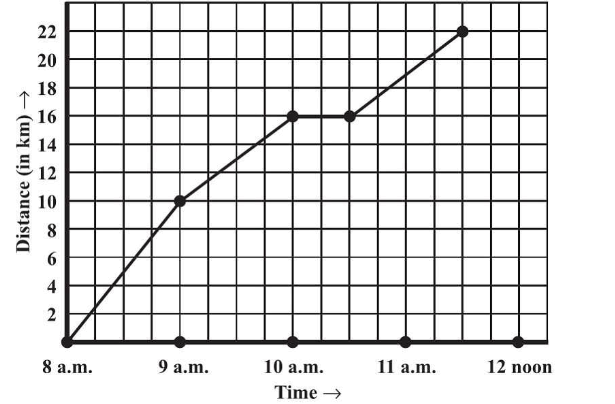# Ex.15.1 Q6 Introduction to Graphs Solutions - NCERT Maths Class 8

Go back to  'Ex.15.1'

## Question

A courier-person cycles from a town to a neighboring suburban area to deliver a parcel to a merchant. His distance from the town at different times is shown by the following graph.(a) What is the scale taken for the time axis?

(b) How much time did the person take for the travel?

(c) How far is the place of the merchant from the town?

(d) Did the person stop on his way? Explain.

(e) During which period did he ride fastest?

## Text Solution

Reasoning:

Horizontal axis represents ‘Time’ and vertical represents ‘Distance’ in $$\rm{km.}$$

We can find the value of ‘Distance’ on vertical axis according to the given value of ‘Time’ on the horizontal axis value or vice versa.

Steps:

(a) Scale taken for the time axis  i.e. x -axis is $$4$$ units $$= 1$$ hour

(b) The person travelled during the time $$8 \;\rm{a.m.} − 11:30 \;\rm{a.m.}$$

Therefore, the person took \begin{align}3\frac{1}{2}\end{align} hours to travel.

(c) The place of the merchant is $$22\,\rm{ km}$$ far from the town.

(d) Yes, the person stopped on his way from $$10\,\rm{ a.m.}$$ to $$10: 30\;\rm{a.m.}$$ This is indicated by the horizontal part of the graph.

(e) From the graph, it can be observed that during $$8 \;\rm{a.m.}$$ to $$9\;\rm{ a.m.},$$ the person travelled the maximum distance. Thus, the person’s ride was the fastest between $$8 \;\rm{a.m.}$$ and $$9 \;\rm{a.m.}$$

Learn from the best math teachers and top your exams

• Live one on one classroom and doubt clearing
• Practice worksheets in and after class for conceptual clarity
• Personalized curriculum to keep up with school